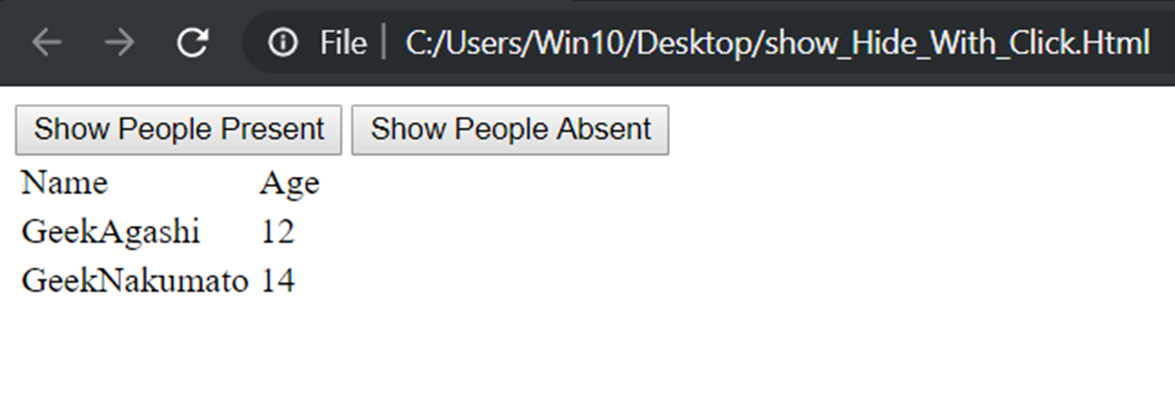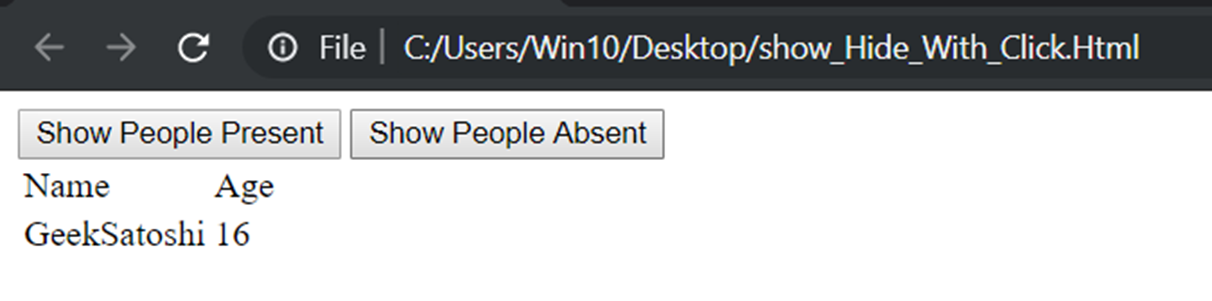# How to hide or show one record from an ng-repeat within a table based on ng-click?

• Difficulty Level : Expert
• Last Updated : 18 Oct, 2019

The way to hide or show a particular record in a table is similar to what is used to hide or show any elements of the DOM. The basic and the first thing that comes to mind is the ng-show and ng-hide directives which show or hides respectively based on binary results of the expressions bound to them. Another way can be the use of
ng-if which works like an if block in general programming. If the expression is true the element is visible or not.
Controlling this can be easily done by the ng-click command which can be used to call a function or run a piece of code that manipulates the entities present in the boolean expressions.

Approach 1: Here the tr element is visible only if the expression bound to the ng-show is true.
Here ng-hide is commented out in the example but it works in the same way ng-show. The difference is that it hides the tr element if the boolean expression gives a true value.
The ng-show and ng-hide directives both can have general function calls but they should return a boolean value.

Syntax:

```< tr ng-repeat="x in [some list]" ng-show="[some boolean expression]" > < tr >
< tr ng-repeat="x in [some list]" ng-hide="[some boolean expression]" > < tr >
```

Example:

 ```<``html``>`` ` `<``head``>``    ``<``title``>``      ``Angular show hide table element on click``  ````    ``<``script` `src``=``"https://ajax.googleapis.com/ajax/libs/angularjs/1.3.14/angular.min.js"``>``    `````` ` `<``body``>``    ``<``div` `ng-app``=``"mainApp"` `ng-controller``=``"MyCtrl"``>``        ``<``button` `ng-click``=``"showPresent()"``>``          ``Show People Present``      ````        ``<``button` `ng-click``=``"showAbsent()"``>``          ``Show People Absent``      ````        ``<``table``>``            ``<``tr``>``                ``<``td``>Name``                ``<``td``>Age``            ````            ````            ````            ``<``tr` `ng-repeat``=``"p in people"` `ng-show``=``"p.attended==flag"``>``                ``<``td``>{{p.name}}``                ``<``td``>{{p.Age}}``            ````        ````    ````    ``<``script``>``        ``var app = angular.module("mainApp", []);``        ``app.controller("MyCtrl", function(\$scope) {``            ``\$scope.flag = 1;``            ``\$scope.people = [{``                ``name: "GeekAgashi",``                ``Age: 12,``                ``attended: 1``            ``}, {``                ``name: "GeekSatoshi",``                ``Age: 16,``                ``attended: 0``            ``}, {``                ``name: "GeekNakumato",``                ``Age: 14,``                ``attended: 1``            ``}];``            ``\$scope.showPresent = function() {``                ``\$scope.flag = 1;``            ``};``            ``\$scope.showAbsent = function() {``                ``\$scope.flag = 0;``            ``};``        ``});``    ```` ` ``` ` ``

Output:

• When the “Show People Present” is clicked the boolean is true for all objects whose attended value matches the flag value as it is changes to 1 by call from ng-click:• When the “Show People Absent” is clicked the boolean is true for all objects whose attended value matches the flag value as it is changes to 0 by call from ng-click:Approach 2: Here we use the ngIf to show or hide records of the table. The code remains the same as the work is nearly the same. But ngIf is less reliable than ng-show or ng-hide but what it does is that it removes the elements from the DOM completely.

Syantax:

`< tr ng-repeat="x in [some list]" ng-if="[some boolean expression]" > < tr >`

Example:

 ```<``html``>`` ` `<``head``>``    ``<``title``>Angular show or hide element on click``    ``<``script` `src``=``"https://ajax.googleapis.com/ajax/libs/angularjs/1.3.14/angular.min.js"``>``    `````` ` `<``body``>``    ``<``div` `ng-app``=``"mainApp"` `ng-controller``=``"MyCtrl"``>``        ``<``button` `ng-click``=``"showPresent()"``>``          ``Show People Present``      ````        ``<``button` `ng-click``=``"showAbsent()"``>``          ``Show People Absent``      ````        ``<``table``>``            ``<``tr``>``                ``<``td``>Name``                ``<``td``>Age``            ````            ````            ``<``tr` `ng-repeat``=``"p in people"``                ``ng-if``=``"p.attended==flag"``>``                ``<``td``>{{p.name}}``                ``<``td``>{{p.Age}}``            ````        ````    ````    ``<``script``>``        ``var app = angular.module("mainApp", []);``        ``app.controller("MyCtrl", function(\$scope) {``            ``\$scope.flag = 1;``            ``\$scope.people = [{``                ``name: "GeekAgashi",``                ``Age: 12,``                ``attended: 1``            ``}, {``                ``name: "GeekSatoshi",``                ``Age: 16,``                ``attended: 0``            ``}, {``                ``name: "GeekNakumato",``                ``Age: 14,``                ``attended: 1``            ``}];``            ``\$scope.showPresent = function() {``                ``\$scope.flag = 1;``            ``};``            ``\$scope.showAbsent = function() {``                ``\$scope.flag = 0;``            ``};``        ``});``    ```` ` ``` ` ``

Output:

• Output remains the same as they both work in the same way:•My Personal Notes arrow_drop_up